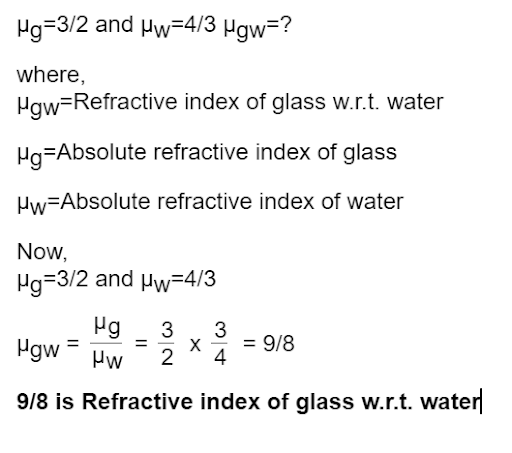# If the absolute refractive indices of glass and water are 3/2 and 4/3 respectively, what is the refractive index of glass with respect to water?

b) if the absolute refractive indices of glass and water are 3/2 and 4/3 respectively, what is the refractive index of glass with respect to water?

verified9/8 is Refractive index of glass w.r.twater